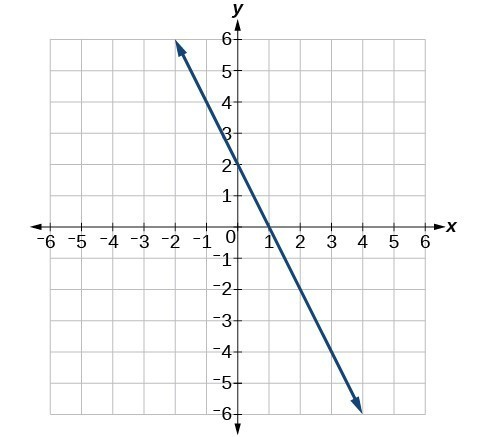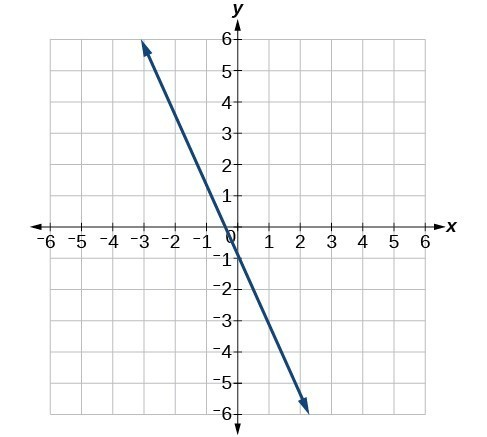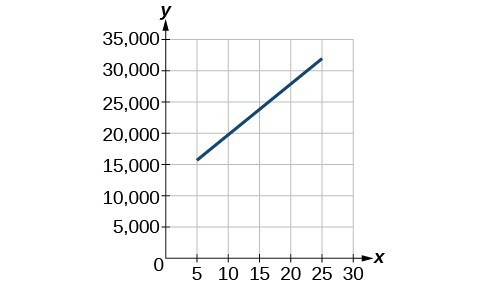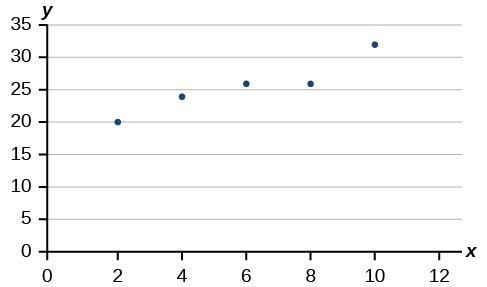## Linear Functions Practice Test

1. Determine whether the following algebraic equation can be written as a linear function. 2+ 3= 7

2. Determine whether the following function is increasing or decreasing. f(x) = –2x + 5

3. Determine whether the following function is increasing or decreasing. f(x) = 7+ 9

4. Given the following set of information, find a linear equation satisfying the conditions, if possible.

Passes through (5, 1) and (3, –9)

5. Given the following set of information, find a linear equation satisfying the conditions, if possible.

x intercept at (–4, 0) and y-intercept at (0, –6)

6. Find the slope of the line in the graph below.7. Write an equation for line in the graph below.8. Does the table below represent a linear function? If so, find a linear equation that models the data.

 x –6 0 2 4 g(x) 14 32 38 44

9. Does the table below represent a linear function? If so, find a linear equation that models the data.

 x 1 3 7 11 g(x) 4 9 19 12

10. At 6 am, an online company has sold 120 items that day. If the company sells an average of 30 items per hour for the remainder of the day, write an expression to represent the number of items that were sold $n$ after 6 am.

For the following exercises, determine whether the lines given by the equations below are parallel, perpendicular, or neither parallel nor perpendicular:

11. $\begin{cases} y=\frac{3}{4}x - 9\hfill \\ -4x - 3y=8\hfill \end{cases}\\$

12. $\begin{cases} -2x+y=3\hfill \\ 3x+\frac{3}{2}y=5\hfill \end{cases}\\$

13. Find the x- and y-intercepts of the equation 2+ 7= –14.

14. Given below are descriptions of two lines. Find the slopes of Line 1 and Line 2. Is the pair of lines parallel, perpendicular, or neither?
Line 1: Passes through (–2, –6) and (3, 14)
Line 2: Passes through (2, 6) and (4, 14)

15. Write an equation for a line perpendicular to f(x) = 4+ 3 and passing through the point (8, 10).

16. Sketch a line with a y-intercept of (0, 5) and slope $-\frac{5}{2}\\$ .

17. Graph the linear function f(x) = –+ 6 .

18. For the two linear functions, find the point of intersection: $\begin{cases}x=y+2\\ 2x - 3y=-1\end{cases}\\$

19. A car rental company offers two plans for renting a car.
Plan A: $25 per day and$0.10 per mile
Plan B: \$40 per day with free unlimited mileage
How many miles would you need to drive for plan B to save you money?

20. Find the area of a triangle bounded by the y axis, the line f(x) = 12 – 4x, and the line perpendicular to f that passes through the origin.

21. A town’s population increases at a constant rate. In 2010 the population was 65,000. By 2012 the population had increased to 90,000. Assuming this trend continues, predict the population in 2018.

22. The number of people afflicted with the common cold in the winter months dropped steadily by 25 each year since 2002 until 2012. In 2002, 8,040 people were inflicted. Find the linear function that models the number of people afflicted with the common cold C as a function of the year, t. When will less than 6,000 people be afflicted?

For the following exercises, use the graph below, showing the profit, y, in thousands of dollars, of a company in a given year, x, where x represents years since 1980.23. Find the linear function y, where y depends on x, the number of years since 1980.

24. Find and interpret the y-intercept.

25. In 2004, a school population was 1250. By 2012 the population had dropped to 875. Assume the population is changing linearly.

a. How much did the population drop between the year 2004 and 2012?
b. What is the average population decline per year?
c. Find an equation for the population, P, of the school t years after 2004.

26. Draw a scatter plot for the data provided below. Then determine whether the data appears to be linearly related.

 0 2 4 6 8 10 –450 –200 10 265 500 755

27. Draw a best-fit line for the plotted data.For the following exercises, use the table below, which shows the percent of unemployed persons 25 years or older who are college graduates in a particular city, by year.

 Year Percent Graduates 2000 8.5 2002 8.0 2005 7.2 2007 6.7 2010 6.4

28. Determine whether the trend appears linear. If so, and assuming the trend continues, find a linear regression model to predict the percent of unemployed in a given year to three decimal places.

29. In what year will the percentage drop below 4%?

30. Based on the set of data given in the table below, calculate the regression line using a calculator or other technology tool, and determine the correlation coefficient. Round to three decimal places of accuracy.

 x 16 18 20 24 26 y 106 110 115 120 125

For the following exercises, consider this scenario: The population of a city increased steadily over a ten-year span. The following ordered pairs shows the population (in hundreds) and the year over the ten-year span, (population, year) for specific recorded years:

(4,500, 2000); (4,700, 2001); (5,200, 2003); (5,800, 2006)

31. Use linear regression to determine a function y, where the year depends on the population. Round to three decimal places of accuracy.

32. Predict when the population will hit 20,000.

33. What is the correlation coefficient for this model?